Next: About this document Up: My Home Page

# AVL Trees Lecture 24

Steven S. Skiena

AVL Trees

An AVL tree is a binary search tree in which the heights of the left and right subtrees of the root differ by at most 1, and the left and right subtrees are again AVL trees.

Therefore, we can label each node of an AVL tree with a balance factor as well as a key:

• ``='' - both subtrees of the node are of equal height
• ``/'' - the left subtree is one taller than the right subtree
• ``
'' - the right subtree is one taller than the left subtree.

AVL trees are named after their inventors, the Russians G.M. Adel'son-Velshi, and E.M. Laudis in 1962.

These are the most unbalanced possible AVL trees with a skew always to the right.

By maintaining the balance of each node (i.e. the subtree below it) when we insert a new node, we can easily see whether or not to take action!

The balance is more useful than maintaining the height of each node because it is a relative, not absolute measure. Thus we can move subtrees around without affecting their balance, even if they end up at different heights.

How good are AVL trees?

To find out how bad they can be, we want to find what the minimum number of modes a tree of height h can have. If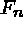is a minimum node AVL tree, its left and right subtrees must themselves be minimum node AVL trees of smaller size. Further, they should differ in height by 1 to take advantage of AVL freedom.

Counting the root node,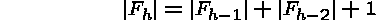Such trees are called Fibonacci trees and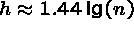.

Thus the worse case AVL tree is almost as good as a random tree - on average it is very close to an optional tree.

Why are Fibonacci trees of logarithmic height?

Recall that the Fibonacci numbers are defined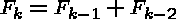,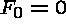,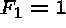.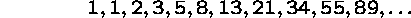Since we are adding the last two numbers together, we are more than doubling the next-to-last and somewhat less that doubling the last number.

In fact,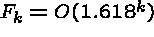, so a tree with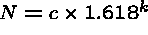nodes has height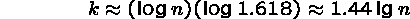AVL Trees Interface

```
INTERFACE AVLTree;          (*08.07.94. CW, LB*)
(* Balanced binary search tree, subtype of "BinaryTree.T" *)

IMPORT BinaryTree;
TYPE T <: BinaryTree.T;   (*T is a subtype of BinaryTree.T *)

END AVLTree.```

AVL Trees Implementation

```MODULE AVLTree EXPORTS AVLTree, AVLTreeRep;  (*08.07.94. CW*)
(* Implementation of the balanced binary search tree as subtype of
"BinaryTree.T". The methods "insert" and "delete" are overwritten
to keep the tree balanced when elements are inserted or
deleted. The other methods are inhereted from the supertype.
*)

IMPORT BinaryTree, BinTreeRep;

REVEAL
T = BinaryTree.T BRANDED OBJECT
OVERRIDES
delete:= Delete;
insert:= Insert;
END;

PROCEDURE Insert(tree: T; e: REFANY) =

PROCEDURE RR (VAR root: BinTreeRep.NodeT) =
(*simple rotation right*)
VAR left:= root.left;
BEGIN
root.left:= left.right;
left.right:= root;
NARROW(root, NodeT).balance:= 0;
root:= left;
END RR;

PROCEDURE RL (VAR root: BinTreeRep.NodeT) =
(*simple rotation left*)
VAR right:= root.right;
BEGIN
root.right:= right.left;
right.left:= root;
NARROW(root, NodeT).balance:= 0;
root:= right;
END RL;

PROCEDURE RrR (VAR root: BinTreeRep.NodeT) =
(*double rotation right*)
VAR right:= root.left.right;
BEGIN
root.left.right:= right.left;
right.left:= root.left;
IF NARROW(right, NodeT).balance = -1
THEN NARROW(root, NodeT).balance:= +1
ELSE NARROW(root, NodeT).balance:= 0
END;
IF NARROW(right, NodeT).balance = +1
THEN NARROW(root.left, NodeT).balance:= -1
ELSE NARROW(root.left, NodeT).balance:= 0
END;
root.left:= right.right;
right.right:= root;
root:= right;
END RrR;

PROCEDURE RrL (VAR root: BinTreeRep.NodeT) =
(*double rotation left*)
VAR left:= root.right.left;
BEGIN
root.right.left:= left.right;
left.right:= root.right;
IF NARROW(left, NodeT).balance = +1
THEN NARROW(root, NodeT).balance:= -1
ELSE NARROW(root, NodeT).balance:= 0
END;
IF NARROW(left, NodeT).balance = -1
THEN NARROW(root.right, NodeT).balance:= +1
ELSE NARROW(root.right, NodeT).balance:= 0
END;
root.right:= left.left;
left.left:= root;
root:= left;
END RrL;

PROCEDURE InsertBal(VAR root: BinTreeRep.NodeT; new: REFANY;
VAR bal: BOOLEAN) =
BEGIN
IF root = NIL
THEN
root:= NEW(NodeT, info:= new, balance:= 0);

ELSIF tree.compare(new, root.info)<0 THEN
InsertBal(root.left, new, bal);
IF NOT bal THEN  (* bal stops recursion*)
WITH done=NARROW(root, NodeT).balance DO
CASE done OF
|+1=> done:= 0; bal:= TRUE;   (*insertion ok*)
| 0=> done:= -1;              (*still balanced, but continue*)
|-1=>
IF NARROW(root.left, NodeT).balance = -1
THEN RR(root)
ELSE RrR(root)
END;
NARROW(root, NodeT).balance:= 0;
bal:= TRUE;            (*after rotation tree ok*)
END; (*CASE*)
END (*WITH*)
END (*IF*)

ELSE
InsertBal(root.right, new, bal);
IF NOT bal THEN    (* bal is set to stop the recurs. adjustm. of balance *)
WITH done=NARROW(root, NodeT).balance DO
CASE done OF
|-1=> done:= 0; bal:= TRUE;   (*insertion ok *)
| 0=> done:= +1;              (*still balanced, but continue*)
|+1=>
IF NARROW(root.right, NodeT).balance = +1
THEN RL(root)
ELSE RrL(root)
END;
NARROW(root, NodeT).balance:= 0;
bal:= TRUE;           (*after rotation tree ok*)
END; (*CASE*)
END (*WITH*)
END (*IF*)
END;
END InsertBal;

VAR balanced:= FALSE;
BEGIN (*Insert*)
InsertBal(tree.root, e, balanced)
END Insert;

PROCEDURE Delete(tree: T; e: REFANY): REFANY =

PROCEDURE RR (VAR root: BinTreeRep.NodeT;
VAR bal: BOOLEAN) =
(*simple rotation right*)
VAR left:= root.left;
BEGIN
root.left:= left.right;
left.right:= root;
IF NARROW(left, NodeT).balance = 0
THEN
NARROW(root, NodeT).balance:= -1;
NARROW(left, NodeT).balance:= +1;
bal:= TRUE;
ELSE
NARROW(root, NodeT).balance:= 0;
NARROW(left, NodeT).balance:= 0; (*depth changed: continue*)
END;
root:= left;
END RR;

PROCEDURE RL (VAR root: BinTreeRep.NodeT;
VAR bal: BOOLEAN) =
(*simple rotation left*)
VAR right:= root.right;
BEGIN
root.right:= right.left;
right.left:= root;
IF NARROW(right, NodeT).balance = 0
THEN
NARROW(root, NodeT).balance:= +1;
NARROW(right, NodeT).balance:= -1;
bal:= TRUE;
ELSE
NARROW(root, NodeT).balance:= 0;
NARROW(right, NodeT).balance:= 0; (*depth changed: continue*)
END;
root:= right;
END RL;

PROCEDURE RrR (VAR root: BinTreeRep.NodeT) =
(*double rotation right*)
VAR right:= root.left.right;
BEGIN
root.left.right:= right.left;
right.left:= root.left;
IF NARROW(right, NodeT).balance = -1
THEN NARROW(root, NodeT).balance:= +1
ELSE NARROW(root, NodeT).balance:= 0
END;
IF NARROW(right, NodeT).balance = +1
THEN NARROW(root.left, NodeT).balance:= -1
ELSE NARROW(root.left, NodeT).balance:= 0
END;
root.left:= right.right;
right.right:= root;
root:= right;
NARROW(right, NodeT).balance:= 0;
END RrR;

PROCEDURE RrL (VAR root: BinTreeRep.NodeT) =
(*double rotation left*)
VAR left:= root.right.left;
BEGIN
root.right.left:= left.right;
left.right:= root.right;
IF NARROW(left, NodeT).balance = +1
THEN NARROW(root, NodeT).balance:= -1
ELSE NARROW(root, NodeT).balance:= 0
END;
IF NARROW(left, NodeT).balance = -1
THEN NARROW(root.right, NodeT).balance:= +1
ELSE NARROW(root.right, NodeT).balance:= 0
END;
root.right:= left.left;
left.left:= root;
root:= left;
NARROW(left, NodeT).balance:= 0;
END RrL;

PROCEDURE BalanceLeft(VAR root: BinTreeRep.NodeT;
VAR bal: BOOLEAN) =
BEGIN
WITH done = NARROW(root, NodeT).balance DO
CASE done OF
|-1=> done:= 0;                 (*new depth: continue*)
| 0=> done:= 1; bal:= TRUE;     (*balanced ->ok*)
|+1=>                           (*balancing needed*)
IF NARROW(root.right, NodeT).balance >= 0
THEN RL(root, bal)
ELSE RrL(root)
END
END (*CASE*)
END (*WITH*)
END BalanceLeft;

PROCEDURE BalanceRight(VAR root: BinTreeRep.NodeT;
VAR bal: BOOLEAN) =
BEGIN
WITH done = NARROW(root, NodeT).balance DO
CASE done OF
|+1=> done:= 0;                 (*new depth: continue*)
| 0=> done:= -1; bal:= TRUE;    (*balanced ->ok*)
|-1=>                           (*balancing needed*)
IF NARROW(root.left, NodeT).balance <= 0
THEN RR(root, bal)
ELSE RrR(root)
END
END (*CASE*)
END (*WITH*)
END BalanceRight;

PROCEDURE DeleteSmallest(VAR root: BinTreeRep.NodeT;
VAR bal: BOOLEAN): REFANY =
VAR deleted: REFANY;
BEGIN
IF root.left = NIL
THEN
deleted:= root.info;
root:= root.right;
RETURN deleted;
ELSE
deleted:= DeleteSmallest(root.left, bal);
IF NOT bal THEN BalanceLeft(root, bal) END;
RETURN deleted;
END;
END DeleteSmallest;

PROCEDURE Delete(VAR root: BinTreeRep.NodeT; elm: REFANY;
VAR bal: BOOLEAN): REFANY =
VAR deleted: REFANY;
BEGIN
IF root = NIL
THEN RETURN NIL

ELSIF tree.compare(root.info, elm)>0 THEN
deleted:= Delete(root.left, elm, bal);
IF deleted # NIL
THEN
IF NOT bal THEN BalanceLeft(root, bal) END;
RETURN deleted;
ELSE RETURN NIL;
END

ELSIF tree.compare(root.info, elm)<0 THEN
deleted:= Delete(root.right, elm, bal);
IF deleted # NIL
THEN
IF NOT bal THEN BalanceRight(root, bal) END;
RETURN deleted;
ELSE RETURN NIL;
END

ELSE
deleted:= root.info;
IF root.left = NIL
THEN
root:= root.right;
ELSIF root.right = NIL THEN
root:= root.left;
ELSE
root.info:= DeleteSmallest(root.right, bal);
IF NOT bal THEN BalanceRight(root, bal) END;
END;
RETURN deleted;
END;
END Delete;

VAR balanced:= FALSE;
BEGIN (*Delete*)
RETURN Delete(tree.root, e, balanced)
END Delete;

BEGIN
END AVLTree.```

Deletion from AVL Trees

We have seen that AVL trees are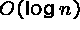for insertion and query.

But what about deletion?

Don't ask! Actually, you can rebalance an AVL tree inbut it is more complicated than insertion.

We will later study B-trees, where deletion is simpler, so don't worry about the details of deletions form AVL trees.Next: About this document Up: My Home Page

Steve Skiena
Tue Nov 25 14:04:19 EST 1997